# Goldberg's conjecture

 Importance: High ✭✭✭
 Author(s): Goldberg, Mark K.
 Subject: Graph Theory » Coloring » » Edge coloring
 Keywords: edge-coloring multigraph
 Posted by: mdevos on: October 4th, 2008

The overfull parameter is defined as follows:Conjecture   Every graphsatisfies.

This important problem remains open despite considerable attention. The same conjecture was independently discovered by Andersen and Seymour.

Vizing's Theorem, one of the cornerstones of graph colouring, shows thatfor every simple graph. So, in particular, every simple graph satisfies Goldberg's conjecture. Graphs with parallel edges need not satisfy Vizing's bound. For instance, ifis the graph obtained from a triangle by adding an extra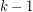edges in parallel with each existing one, thenbut. More generally, ifis a subgraph of, then every colour can appear on at mostedges of, so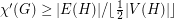. Thus,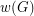, our overfull parameter, is a natural lower bound on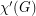, and Goldberg's conjecture asserts that wheneverexceeds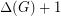, then it is equal to this lower bound.

Although the statement of the conjecture may appear to be the most natural formulation, there are a couple of related conjectures with similar lower bounds. For instance, Seymour's r-graph conjecture is equivalent to the statement that. Goldberg also conjectured that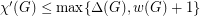.

In addition to simple graphs, Goldberg's Conjecture is known to hold for any graphwhich satisfies one of the following

\item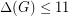\itemhas no minor isomorphic to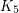minus an edge. \item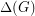is sufficiently large in comparison with.## Bibliography

*[G] M. K. Goldberg, Multigraphs with a chromatic index that is nearly maximal. (Russian) A collection of articles dedicated to the memory of Vitaliĭ Konstantinovič Korobkov. Diskret. Analiz No. 23 (1973), 3--7, 72. MathSciNet

* indicates original appearance(s) of problem.

### Latest Developments

Stiebitz et al(2006), Yu(2008) and Kurt(2009) has separately shown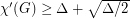implies Goldberg Conjecture. While Yu's method gives a methodological approach to the general problem, Kurt provides a very short and elementary proof.

Scheide (2008) has shown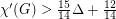implies the Goldberg Conjecture.

Kurt(2009) has shown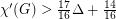implies the Goldberg Conjecture.

### Different Goldberg Conjecture?

http://plms.oxfordjournals.org/content/106/4/703

--Stephen